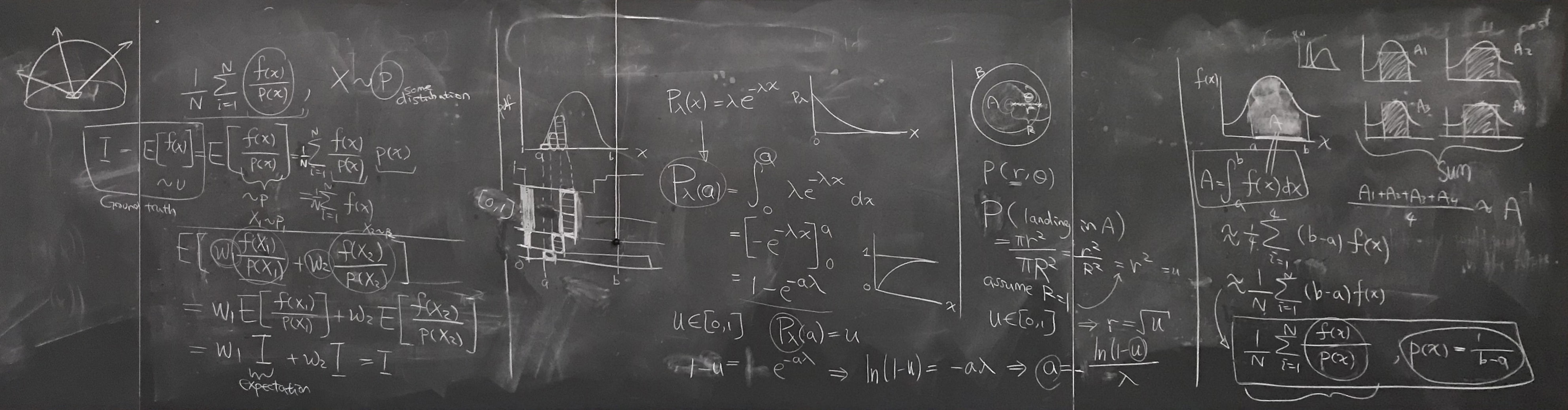Discussion 6

Bullets

• Monte Carlo integration
• "Curse of dimensionality"
• Monte Carlo estimator $F_N = \frac{1}{N} \sum_{i=1}^{N} \frac{f(X_i)}{p(X_i)}$
• Importance sampling
• Draw samples from desired distribution
• Inverse CDF
• Draw uniform samples from circle

🖍 Board work for everythingBoard work at the end of section